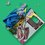# Attempting to derive a formula for logistic map (part 1)

The logistic map comes from concatenations of the function $f(x) = \lambda x(1-x)$, where $x=\frac12$ and$\lambda$ is an argument within the domain $[0,4]$. We'll begin by defining a function $f_n (x)$ as $n$ instances of $f(x)$ concatenated together, observing the fractional form of each incrementation of $n$: $f_1 \left(\frac12\right) = \lambda \frac12 \left(1-\frac12\right) = \frac{\lambda}4$ $f_2 \left(\frac12\right) = \lambda \frac{\lambda}4 \left(1-\frac{\lambda}4\right) = \frac{\lambda^2 (4-\lambda)}{16}$ $f_3 \left(\frac12\right) = \lambda \frac{\lambda^2 (4-\lambda)}{16} \left(1-\frac{\lambda^2 (4-\lambda)}{16} \right) = \frac{\lambda^3(4-\lambda)(16-\lambda^2(4-\lambda))}{256}$ The denominator of each iteration is the square of the previous denominator. Using the fact that $4$ is $2^{2^1}$, we can rewrite $f_n \left(\frac12\right) = \frac{f_n^{'}\left(\frac12\right)}{2^{2^n}}$ (here $f_n^{'}(x)$ just denotes the remaining sections of $f_n (x)$.)
Note that the leading power of $\lambda$ in each term cannot be taken out in this state, due to it being concentated into $f_{n+1}$. However, this option may be feasible within sums of $\lambda^{n-k}$. To derive bounds for each power, we can: $f_1^{'} \left(\frac12\right) = \lambda$ $f_2^{'} \left(\frac12\right) = \lambda^2 (4-\lambda) = 4\lambda^2-\lambda^3$ $f_3^{'} \left(\frac12\right) =\lambda(4\lambda^2-\lambda^3)(16-4\lambda^2+\lambda^3)= 64\lambda^3-16\lambda^5+4\lambda^6-16\lambda^4+4\lambda^6-\lambda^7$$= 64\lambda^3-16\lambda^4-16\lambda^5+8\lambda^6-\lambda^7$ The lowest order $k$ of $\lambda^k$ is $n$, as a constant times $\lambda$ times $\lambda^{n-1}$, and the highest order is $2^n-1$, as $\left(\lambda^{2^{n-1}-1}\right)^2$ times $\lambda$ produces $\lambda^{2(2^{n-1}-1)+1}=\lambda^{2^n-1}$. Given the coefficients $c_{n,k}$, we can express $f_n^{'}(x)$ as $\sum_{k=0}^{2^n-n-1} c_{n,k} x^{k+n}$ More iterations of $n$ will be needed to figure out a general formula for these coefficients.Note by William Crabbe
3 years ago

This discussion board is a place to discuss our Daily Challenges and the math and science related to those challenges. Explanations are more than just a solution — they should explain the steps and thinking strategies that you used to obtain the solution. Comments should further the discussion of math and science.

When posting on Brilliant:

• Use the emojis to react to an explanation, whether you're congratulating a job well done , or just really confused .
• Ask specific questions about the challenge or the steps in somebody's explanation. Well-posed questions can add a lot to the discussion, but posting "I don't understand!" doesn't help anyone.
• Try to contribute something new to the discussion, whether it is an extension, generalization or other idea related to the challenge.

MarkdownAppears as
*italics* or _italics_ italics
**bold** or __bold__ bold
- bulleted- list
• bulleted
• list
1. numbered2. list
1. numbered
2. list
Note: you must add a full line of space before and after lists for them to show up correctly
paragraph 1paragraph 2

paragraph 1

paragraph 2

[example link](https://brilliant.org)example link
> This is a quote
This is a quote
    # I indented these lines
# 4 spaces, and now they show
# up as a code block.

print "hello world"
# I indented these lines
# 4 spaces, and now they show
# up as a code block.

print "hello world"
MathAppears as
Remember to wrap math in $$ ... $$ or $ ... $ to ensure proper formatting.
2 \times 3 $2 \times 3$
2^{34} $2^{34}$
a_{i-1} $a_{i-1}$
\frac{2}{3} $\frac{2}{3}$
\sqrt{2} $\sqrt{2}$
\sum_{i=1}^3 $\sum_{i=1}^3$
\sin \theta $\sin \theta$
\boxed{123} $\boxed{123}$